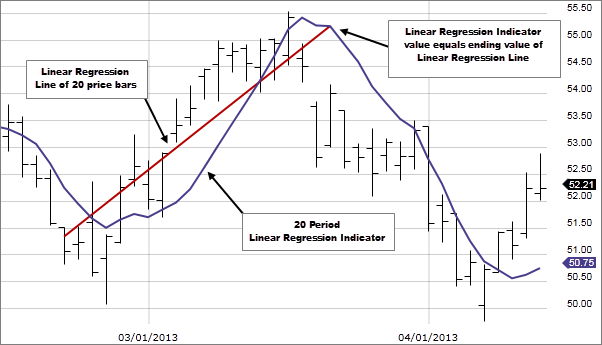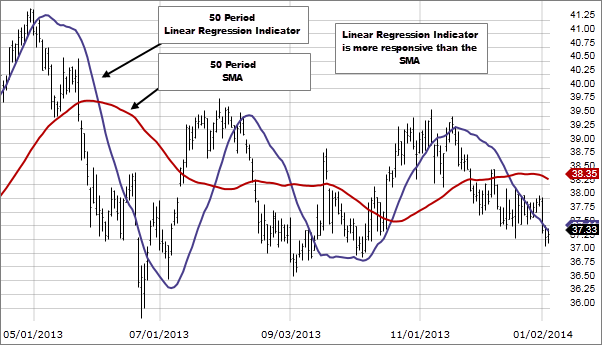# Linear Regression

### Description

The Linear Regression Indicator plots the ending value of a Linear Regression Line for a specified number of bars; showing, statistically, where the price is expected to be. For example, a 20 period Linear Regression Indicator will equal the ending value of a Linear Regression line that covers 20 bars.### How this indicator works

• The interpretation of the Linear Regression Indicator is similar to that of a moving average although it does have an advantage when compared to a moving average.
• Instead of plotting an average of past price action, it is plotting where a Linear Regression Line would expect the price to be, making the Linear Regression Indicator more responsive than a moving average.### Calculation

Each point in the Linear Regression Indicator is simply the end-point of an n-period Linear Regression trendline.

Technical analysis focuses on market action — specifically, volume and price. Technical analysis is only one approach to analyzing stocks. When considering which stocks to buy or sell, you should use the approach that you're most comfortable with. As with all your investments, you must make your own determination as to whether an investment in any particular security or securities is right for you based on your investment objectives, risk tolerance, and financial situation. Past performance is no guarantee of future results.

676794.3.0